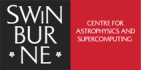## SAO Mathematical Requirements

Astronomy is based on mathematics. Our introductory units focus on a conceptual approach to astronomy but will at times require some basic mathematics. Advanced SAO units, however, do require some background mathematical experience.

Mathematics Background

The introductory-level units, AST80005 Exploring the Solar System, AST80004 Exploring Stars & the Milky Way, AST80006 Galaxies and their Place in the Universe and AST80008 History of Astronomy, may require some basic maths (i.e via our newsgroup discussions, and as part of Computer Managed Tests). The more advanced-level units AST80003 Cosmology and the Large-scale Structure of the Universe, AST80016 Stellar Astrophysics and AST80011 Major Project: Computational Astrophysics, assume some background mathematical knowledge. The following summary is a good guide for advanced-level units currently being offered:

• Basic Algebra:
rules of algebra, functions, equations, cartesian coordinates, linear equations, graphs of simple equations, exponential functions & logarithms
• Basic Geometry & Trigonometry:
straight lines, circles, ellipses, parabolas, spheres, definition & manipulation of simple trigonometric functions such as sine, cosine & tangent
• Calculus:
graphical interpretation of differentiation & integration, eg. rate of change, instantaneous slope of a curve & differentiation, area under a curve & integration (integration & differentiation of mathematical expressions not required)

If other maths concepts & techniques are required, we will teach them as part of our course material.

Note that all SAO course material contain some mathematical equations - this is astronomy after all! Similarly, some questions in the computer managed tests that form part of the assessment may contain simple maths questions. These will usually be algebra of the type presented in the course material.

Bridging Mathematics

Course participants wishing to "brush up" their mathematics can use the above mathematics topics as a guide, and study from any suitable text.

As an alternative, for self-paced revision we can recommend:

MathCasts: MathsCasts are produced by the mathematics support centres at Swinburne University, the University of Limerick and Loughborough University. They are part of an ongoing collaborative research project to develop high quality resources and investigate the effectiveness of MathsCasts to support mathematics learning. They are targeted at prerequisite to first year level, in a range of subjects such as: Engineering, Sciences, Business, Computing and Technology.

For more information go to Swinburne Commons or search for "MathsCasts" in iTunes.

UNILEARN Senior Mathematics, Modules 1 to 3, a series of bridging mathematics workbooks published by the UNILEARN Learning Network Queensland. The topic of Module 1 is Introduction to Functions, Module 2 covers Coordinate Geometry & Trigonometry, and Module 3 introduces Calculus & its Applications. These Modules somewhat exceed the mathematics requirements listed above, particularly in the area of calculus, but they do provide a well thought-out learning program for bridging mathematics.

Each Module costs \$AU 32.00 plus postage and handling. Note that some of the exercises in these Modules assume access to a graphics calculator. Swinburne University does not supply these Modules or provide further information about them. To order the Senior Mathematics Material from UNILEARN Learning Network Queensland, please fill in the UNILEARN materials order form. If you would like further information, visit www.unilearn.net.au or contact:

Dr. Jenelle Benson, LVT Unilearn  Email: J.Benson@LNQ.net.au Phone: +61 7 3307 4775 Fax: +61 7 3869 8274 Post: Unilearn Learning Network Queensland Locked Mail Bag 3 Eagle Farm BC QLD 4009 Australia